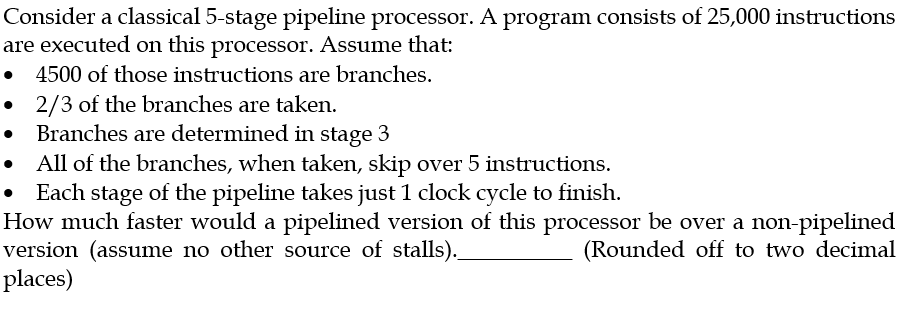104 views### 1 comment

moved ago
Given ,No of instruction =$25000$

No of branch instruction =$4500$

$\frac{2}{3}$ of the branches are taken So no of branch taken = $\frac{2}{3}*4500=3000$ .

If branch taken it will skip 5 instruction for this program .So ,with $3000$ branch instruction that taken , this program will skip =$5*3000=15000$ instruction .

So no of instruction it will execute =$25000-15000=10000$ .

Now the non pipeline and pipeline processor both will execute only $10000$ instruction .

Branch is determined in stage 3 so branch penalty=2 stall cycle.

So , no of clock cycle required to execute in pipeline =$10000*1+2*3000=16000$

No of clock cycle required to execute it in non pipeline $=5*10000=50000$

So, Speed up=$\frac{50000}{16000}=3.125$  [assuming clock cycle time for both pipeline and non pipeline are same]

1
110 views